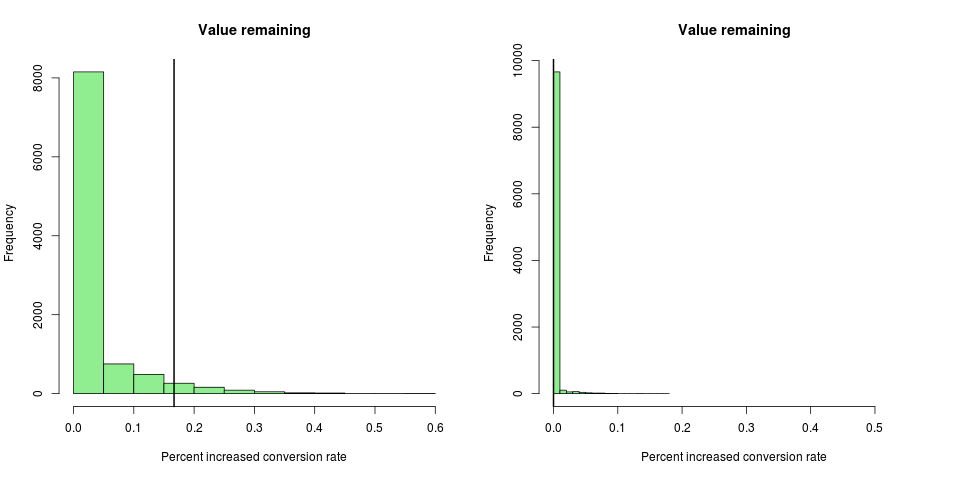# Appendix (multi-armed bandit)

Computational and theoretical details

First, keep in mind that the name “multi-armed bandit” describes a problem to which several “solutions” have been proposed. If you pick up a book on “Reinforcement Learning” you will find several approaches listed in the introductory chapter on multi-armed bandits. This is because the math behind the multi-armed bandit problem is so hard that approximate heuristic solutions are used in practice. The mathematical difficulties are neatly summarized in a famous quote by Peter Whittle (Whittle, 1979):

[The bandit problem] was formulated during the [second world] war, and efforts to solve it so sapped the energies and minds of Allied analysts that the suggestion was made that the problem be dropped over Germany, as the ultimate instrument of intellectual sabotage.

We use a heuristic known as Thompson Sampling, or Randomized Probability Matching, because it combines many of the best features of these heuristics. You can learn more about this technique in , and see more details about its mathematical properties in  , , and .

#### Optimal arm probabilities

Thompson sampling assigns sessions to arms in proportion to the probability that each arm is optimal. This is a Bayesian computation. Let θ = (θ1, θ2,..., θk) denote the vector of conversion rates for arms 1, …, k. And let y denote the data observed thus far in the experiment. We model y as a vector of independent binomial outcomes and assume independent uniform priors on θ. Let Ia(θ) denote the indicator of the event that arm a is optimal. Then we can write:

P(Ia) = ∫Ia(θ) p(θ|y) dθ

This integral can be done in closed form (although the closed form solution involves complicated special functions like the incomplete beta function), or by numerical integration. In either case, the computation quickly becomes unstable even for relatively small values of y. However, optimal arm probabilities can be stably computed by simulation. Each element of θ is an independent random variable from the beta distribution. Simulate a large matrix containing draws of θ from the relevant beta distributions, where the rows of the matrix represent random draws, and the columns represent the k arms of the experiment. A Monte Carlo estimate of the probability that arm a is optimal is the empirical fraction of rows for which arm a had the largest simulated value. The probability that each arm beats the original can be computed similarly.

#### Value remaining

The simulation that produces the optimal-arm probabilities can also produce the distribution of the value remaining in the experiment. The value remaining is the posterior distribution of (θmax-θ*)/θ*, where θmax is the largest value of θ, and θ* is the value of θ for the arm that is most likely to be optimal. To illustrate the computation, suppose there are three arms with 20, 30, and 40 sessions that have generated 12, 20, and 30 conversions. The optimal arm probabilities are roughly .09, .20, and .71. The first 6 draws from the Monte Carlo simulation of θ might be:

 [,1] [,2] [,3] [1,] 0.54 0.73 0.74 [2,] 0.55 0.66 0.73 [3,] 0.53 0.81 0.80 [4,] 0.57 0.50 0.65 [5,] 0.52 0.67 0.83 [6,] 0.65 0.84 0.63

We compute value row by row by subtracting the largest element of that row from the element in column 3 (because arm 3 has the highest chance of being the optimal arm). In the first two rows the value is zero because the largest draw occurs in column 3. In the third row the value is .01/.80 because column 2 is .01 larger than column 3. If we keep going down each row we get a distribution of values that we could plot in a histogram like the left panel of Figure A1. Arm 3 has a 71% probability of being the best arm, so the value of switching away from arm 3 is zero in 71% of the cases. The 95th percentile of the value distribution is the “potential value remaining” in the experiment, which in this case works out to be about .16. You interpret this number as “We’re still unsure about the CvR for arm 3, but whatever it is, one of the other arms might beat it by as much as 16%.”

The right panel in Figure A1 shows what happens to the value-remaining distribution as the experiment progresses. Suppose each arm had 5 times the sample size (so 100, 150, and 200 sessions), with 5 times the number of conversions (60, 100, 150). With larger sample sizes, we are much more confident about the conversion rates of the arms. Arm 3 now has about a 95% chance of being the optimal arm, so the 95th percentile of the value-remaining distribution is zero.Figure A1. The distribution of the value remaining in an experiment. The vertical line in each case is the 95th percentile, or the potential value remaining.